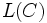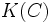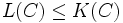# Difference between revisions of "Fary's theorem"

Let$C$ be a closed curve lying inside a unit disc. Then, if$L(C)$ denotes the length of$C$ and$K(C)$ denotes the total curvature of$C$:$L(C) \le K(C)$